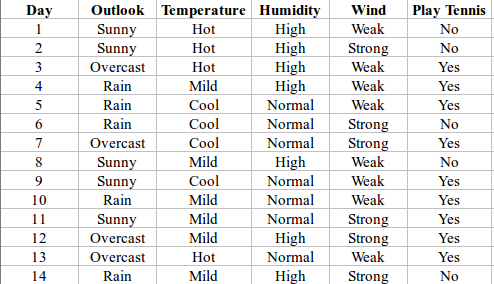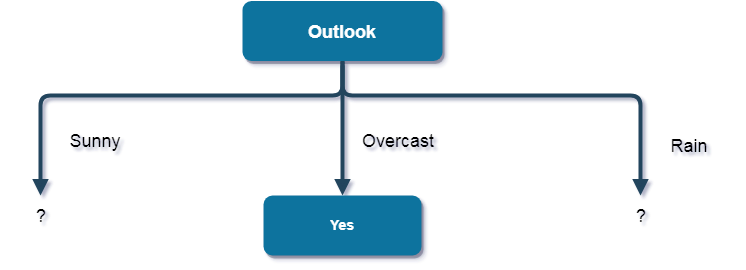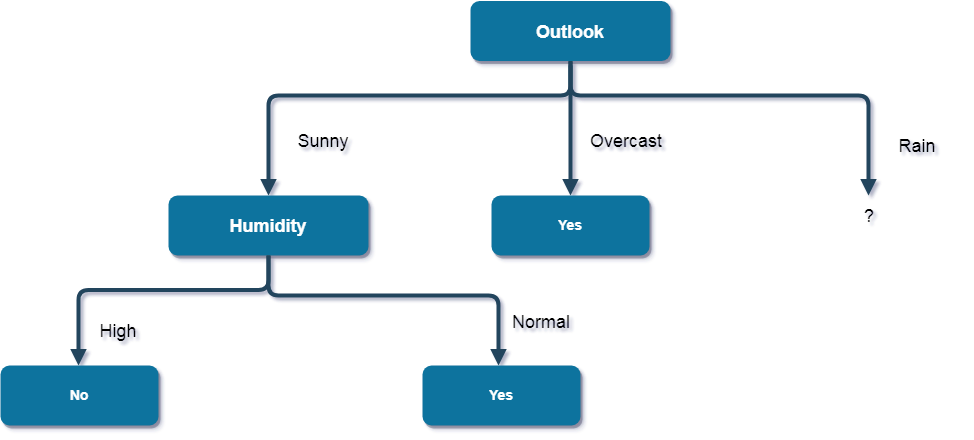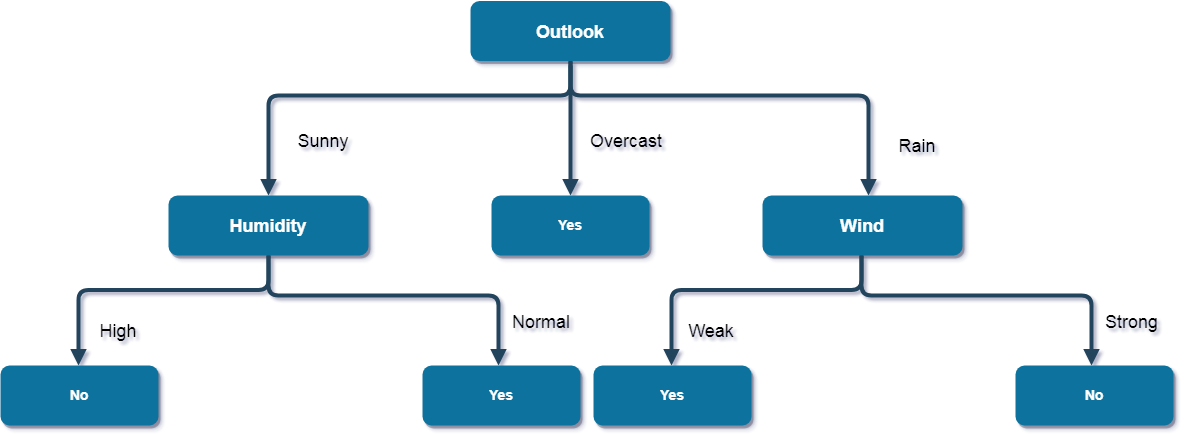×

Search anything:

# Using ID3 Algorithm to build a Decision Tree to predict the weather

#### Machine Learning (ML) data mining decision tree id3 algorithmGet this book -> Problems on Array: For Interviews and Competitive Programming

ID3 algorithm, stands for Iterative Dichotomiser 3, is a classification algorithm that follows a greedy approach of building a decision tree by selecting a best attribute that yields maximum Information Gain (IG) or minimum Entropy (H).

In this article, we will use the ID3 algorithm to build a decision tree based on a weather data and illustrate how we can use this procedure to make a decision on an action (like whether to play outside) based on the current data using the previously collected data.

We will go through the basics of decision tree, ID3 algorithm before applying it to our data. We will answer the following questions:

• What is a decision tree?
• What is ID3 algorithm?
• What is information gain and entropy?
• What are the steps in ID3 algorithm?
• Using ID3 algorithm on a real data
• What are the characteristics of ID3 algorithm?

# What is a Decision Tree?

A Supervised Machine Learning Algorithm, used to build classification and regression models in the form of a tree structure.

A decision tree is a tree where each -

• Node - a feature(attribute)
• Branch - a decision(rule)
• Leaf - an outcome(categorical or continuous)

There are many algorithms to build decision trees, here we are going to discuss ID3 algorithm with an example.

# What is an ID3 Algorithm?

ID3 stands for Iterative Dichotomiser 3

It is a classification algorithm that follows a greedy approach by selecting a best attribute that yields maximum Information Gain(IG) or minimum Entropy(H).

## What is Entropy and Information gain?

Entropy is a measure of the amount of uncertainty in the dataset S. Mathematical Representation of Entropy is shown here -

$H\left(S\right)=\sum _{c\in C}-p\left(c\right)lo{g}_{2}p\left(c\right)$

Where,

• S - The current dataset for which entropy is being calculated(changes every iteration of the ID3 algorithm).
• C - Set of classes in S {example - C ={yes, no}}
• p(c) - The proportion of the number of elements in class c to the number of elements in set S.

In ID3, entropy is calculated for each remaining attribute. The attribute with the smallest entropy is used to split the set S on that particular iteration.

Entropy = 0 implies it is of pure class, that means all are of same category.

Information Gain IG(A) tells us how much uncertainty in S was reduced after splitting set S on attribute A. Mathematical representation of Information gain is shown here -

$IG\left(A,S\right)=H\left(S\right)-\sum _{t\in T}p\left(t\right)H\left(t\right)$

Where,

• H(S) - Entropy of set S.
• T - The subsets created from splitting set S by attribute A such that
$S=\bigcup _{tϵT}t$
• p(t) - The proportion of the number of elements in t to the number of elements in set S.
• H(t) - Entropy of subset t.

In ID3, information gain can be calculated (instead of entropy) for each remaining attribute. The attribute with the largest information gain is used to split the set S on that particular iteration.

# What are the steps in ID3 algorithm?

The steps in ID3 algorithm are as follows:

1. Calculate entropy for dataset.
2. For each attribute/feature.
2.1. Calculate entropy for all its categorical values.
2.2. Calculate information gain for the feature.
3. Find the feature with maximum information gain.
4. Repeat it until we get the desired tree.

# Use ID3 algorithm on a data

We'll discuss it here mathematically and later see it's implementation in Python.
So, Let's take an example to make it more clear.Here,dataset is of binary classes(yes and no), where 9 out of 14 are "yes" and 5 out of 14 are "no".

Complete entropy of dataset is:

``````H(S) = - p(yes) * log2(p(yes)) - p(no) * log2(p(no))
= - (9/14) * log2(9/14) - (5/14) * log2(5/14)
= - (-0.41) - (-0.53)
= 0.94
``````

For each attribute of the dataset, let's follow the step-2 of pseudocode : -

## First Attribute - Outlook

``````Categorical values - sunny, overcast and rain
H(Outlook=sunny) = -(2/5)*log(2/5)-(3/5)*log(3/5) =0.971
H(Outlook=rain) = -(3/5)*log(3/5)-(2/5)*log(2/5) =0.971
H(Outlook=overcast) = -(4/4)*log(4/4)-0 = 0

Average Entropy Information for Outlook -
I(Outlook) = p(sunny) * H(Outlook=sunny) + p(rain) * H(Outlook=rain) + p(overcast) * H(Outlook=overcast)
= (5/14)*0.971 + (5/14)*0.971 + (4/14)*0
= 0.693

Information Gain = H(S) - I(Outlook)
= 0.94 - 0.693
= 0.247
``````

I suggest you to do the same calculations for all the remaining attributes without scrolling down....

### Second Attribute - Temperature

``````Categorical values - hot, mild, cool
H(Temperature=hot) = -(2/4)*log(2/4)-(2/4)*log(2/4) = 1
H(Temperature=cool) = -(3/4)*log(3/4)-(1/4)*log(1/4) = 0.811
H(Temperature=mild) = -(4/6)*log(4/6)-(2/6)*log(2/6) = 0.9179
Average Entropy Information for Temperature -
I(Temperature) = p(hot)*H(Temperature=hot) + p(mild)*H(Temperature=mild) + p(cool)*H(Temperature=cool)
= (4/14)*1 + (6/14)*0.9179 + (4/14)*0.811
= 0.9108

Information Gain = H(S) - I(Temperature)
= 0.94 - 0.9108
= 0.0292
``````

### Third Attribute - Humidity

``````Categorical values - high, normal
H(Humidity=high) = -(3/7)*log(3/7)-(4/7)*log(4/7) = 0.983
H(Humidity=normal) = -(6/7)*log(6/7)-(1/7)*log(1/7) = 0.591

Average Entropy Information for Humidity -
I(Humidity) = p(high)*H(Humidity=high) + p(normal)*H(Humidity=normal)
= (7/14)*0.983 + (7/14)*0.591
= 0.787

Information Gain = H(S) - I(Humidity)
= 0.94 - 0.787
= 0.153
``````

### Fourth Attribute - Wind

``````Categorical values - weak, strong
H(Wind=weak) = -(6/8)*log(6/8)-(2/8)*log(2/8) = 0.811
H(Wind=strong) = -(3/6)*log(3/6)-(3/6)*log(3/6) = 1

Average Entropy Information for Wind -
I(Wind) = p(weak)*H(Wind=weak) + p(strong)*H(Wind=strong)
= (8/14)*0.811 + (6/14)*1
= 0.892

Information Gain = H(S) - I(Wind)
= 0.94 - 0.892
= 0.048
``````

Here, the attribute with maximum information gain is Outlook. So, the decision tree built so far -Here, when Outlook == overcast, it is of pure class(Yes).
Now, we have to repeat same procedure for the data with rows consist of Outlook value as Sunny and then for Outlook value as Rain.

I again recommend you to perform further calculations and then cross check by scrolling down...

Now, finding the best attribute for splitting the data with Outlook=Sunny values{ Dataset rows = [1, 2, 8, 9, 11]}.

``````Complete entropy of Sunny is -
H(S) = - p(yes) * log2(p(yes)) - p(no) * log2(p(no))
= - (2/5) * log2(2/5) - (3/5) * log2(3/5)
= 0.971
``````

### First Attribute - Temperature

``````Categorical values - hot, mild, cool
H(Sunny, Temperature=hot) = -0-(2/2)*log(2/2) = 0
H(Sunny, Temperature=cool) = -(1)*log(1)- 0 = 0
H(Sunny, Temperature=mild) = -(1/2)*log(1/2)-(1/2)*log(1/2) = 1
Average Entropy Information for Temperature -
I(Sunny, Temperature) = p(Sunny, hot)*H(Sunny, Temperature=hot) + p(Sunny, mild)*H(Sunny, Temperature=mild) + p(Sunny, cool)*H(Sunny, Temperature=cool)
= (2/5)*0 + (1/5)*0 + (2/5)*1
= 0.4

Information Gain = H(Sunny) - I(Sunny, Temperature)
= 0.971 - 0.4
= 0.571
``````

### Second Attribute - Humidity

``````Categorical values - high, normal
H(Sunny, Humidity=high) = - 0 - (3/3)*log(3/3) = 0
H(Sunny, Humidity=normal) = -(2/2)*log(2/2)-0 = 0

Average Entropy Information for Humidity -
I(Sunny, Humidity) = p(Sunny, high)*H(Sunny, Humidity=high) + p(Sunny, normal)*H(Sunny, Humidity=normal)
= (3/5)*0 + (2/5)*0
= 0

Information Gain = H(Sunny) - I(Sunny, Humidity)
= 0.971 - 0
= 0.971
``````

### Third Attribute - Wind

``````Categorical values - weak, strong
H(Sunny, Wind=weak) = -(1/3)*log(1/3)-(2/3)*log(2/3) = 0.918
H(Sunny, Wind=strong) = -(1/2)*log(1/2)-(1/2)*log(1/2) = 1

Average Entropy Information for Wind -
I(Sunny, Wind) = p(Sunny, weak)*H(Sunny, Wind=weak) + p(Sunny, strong)*H(Sunny, Wind=strong)
= (3/5)*0.918 + (2/5)*1
= 0.9508

Information Gain = H(Sunny) - I(Sunny, Wind)
= 0.971 - 0.9508
= 0.0202
``````

Here, the attribute with maximum information gain is Humidity. So, the decision tree built so far -Here, when Outlook = Sunny and Humidity = High, it is a pure class of category "no". And When Outlook = Sunny and Humidity = Normal, it is again a pure class of category "yes". Therefore, we don't need to do further calculations.

Now, finding the best attribute for splitting the data with Outlook=Sunny values{ Dataset rows = [4, 5, 6, 10, 14]}.

``````Complete entropy of Rain is -
H(S) = - p(yes) * log2(p(yes)) - p(no) * log2(p(no))
= - (3/5) * log(3/5) - (2/5) * log(2/5)
= 0.971
``````

### First Attribute - Temperature

``````Categorical values - mild, cool
H(Rain, Temperature=cool) = -(1/2)*log(1/2)- (1/2)*log(1/2) = 1
H(Rain, Temperature=mild) = -(2/3)*log(2/3)-(1/3)*log(1/3) = 0.918
Average Entropy Information for Temperature -
I(Rain, Temperature) = p(Rain, mild)*H(Rain, Temperature=mild) + p(Rain, cool)*H(Rain, Temperature=cool)
= (2/5)*1 + (3/5)*0.918
= 0.9508

Information Gain = H(Rain) - I(Rain, Temperature)
= 0.971 - 0.9508
= 0.0202
``````

### Second Attribute - Wind

``````Categorical values - weak, strong
H(Wind=weak) = -(3/3)*log(3/3)-0 = 0
H(Wind=strong) = 0-(2/2)*log(2/2) = 0

Average Entropy Information for Wind -
I(Wind) = p(Rain, weak)*H(Rain, Wind=weak) + p(Rain, strong)*H(Rain, Wind=strong)
= (3/5)*0 + (2/5)*0
= 0

Information Gain = H(Rain) - I(Rain, Wind)
= 0.971 - 0
= 0.971
``````

Here, the attribute with maximum information gain is Wind. So, the decision tree built so far -Here, when Outlook = Rain and Wind = Strong, it is a pure class of category "no". And When Outlook = Rain and Wind = Weak, it is again a pure class of category "yes".
And this is our final desired tree for the given dataset.

# What are the characteristics of ID3 algorithm?

Finally, I am concluding with Characteristics of ID3.

Characteristics of ID3 Algorithm are as follows:

1. ID3 uses a greedy approach that's why it does not guarantee an optimal solution; it can get stuck in local optimums.
2. ID3 can overfit to the training data (to avoid overfitting, smaller decision trees should be preferred over larger ones).
3. This algorithm usually produces small trees, but it does not always produce the smallest possible tree.
4. ID3 is harder to use on continuous data (if the values of any given attribute is continuous, then there are many more places to split the data on this attribute, and searching for the best value to split by can be time consuming).

With this technical article at OpenGenus, you must have a strong idea of ID3 Algorithm and how to use ID3 Algorithm to build a Decision Tree to predict the weather.

# Hope You enjoyed it!#### Nidhi Mantri

Specialist Programmer at Infosys Ltd; Completed B. Tech in CS at IIIT Kota (2020); Machine Learning certification from deeplearning.ai; Project on Emotion Classification using CNN.

Using ID3 Algorithm to build a Decision Tree to predict the weather